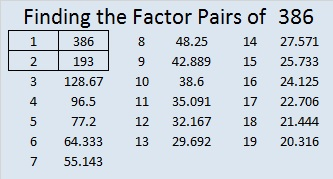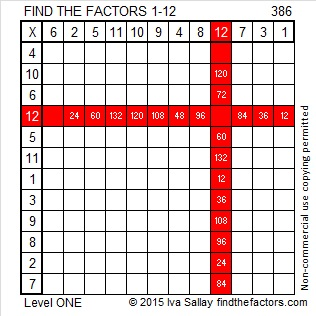# 386 and Level 1

386 is even and composite.Print the puzzles or type the factors on this excel file:12 Factors 2015-02-09

• 386 is a composite number.
• Prime factorization: 386 = 2 x 193
• The exponents in the prime factorization are 1 and 1. Adding one to each and multiplying we get (1 + 1)(1 + 1) = 2 x 2 = 4. Therefore 386 has exactly 4 factors.
• Factors of 386: 1, 2, 193, 386
• Factor pairs: 386 = 1 x 386 or 2 x 193
• 386 has no square factors that allow its square root to be simplified. √386 ≈ 19.647